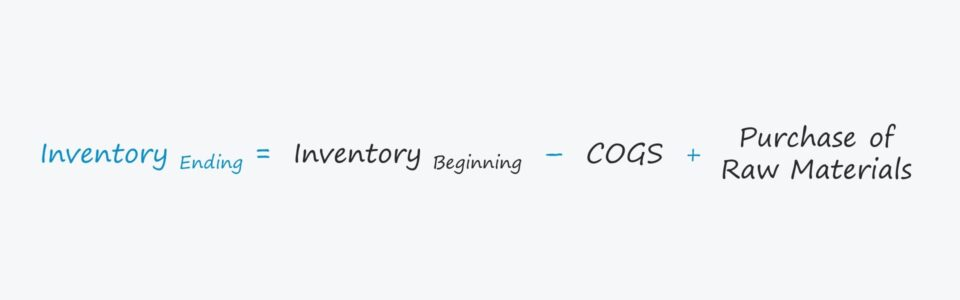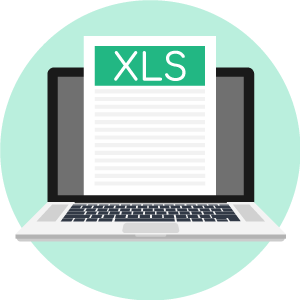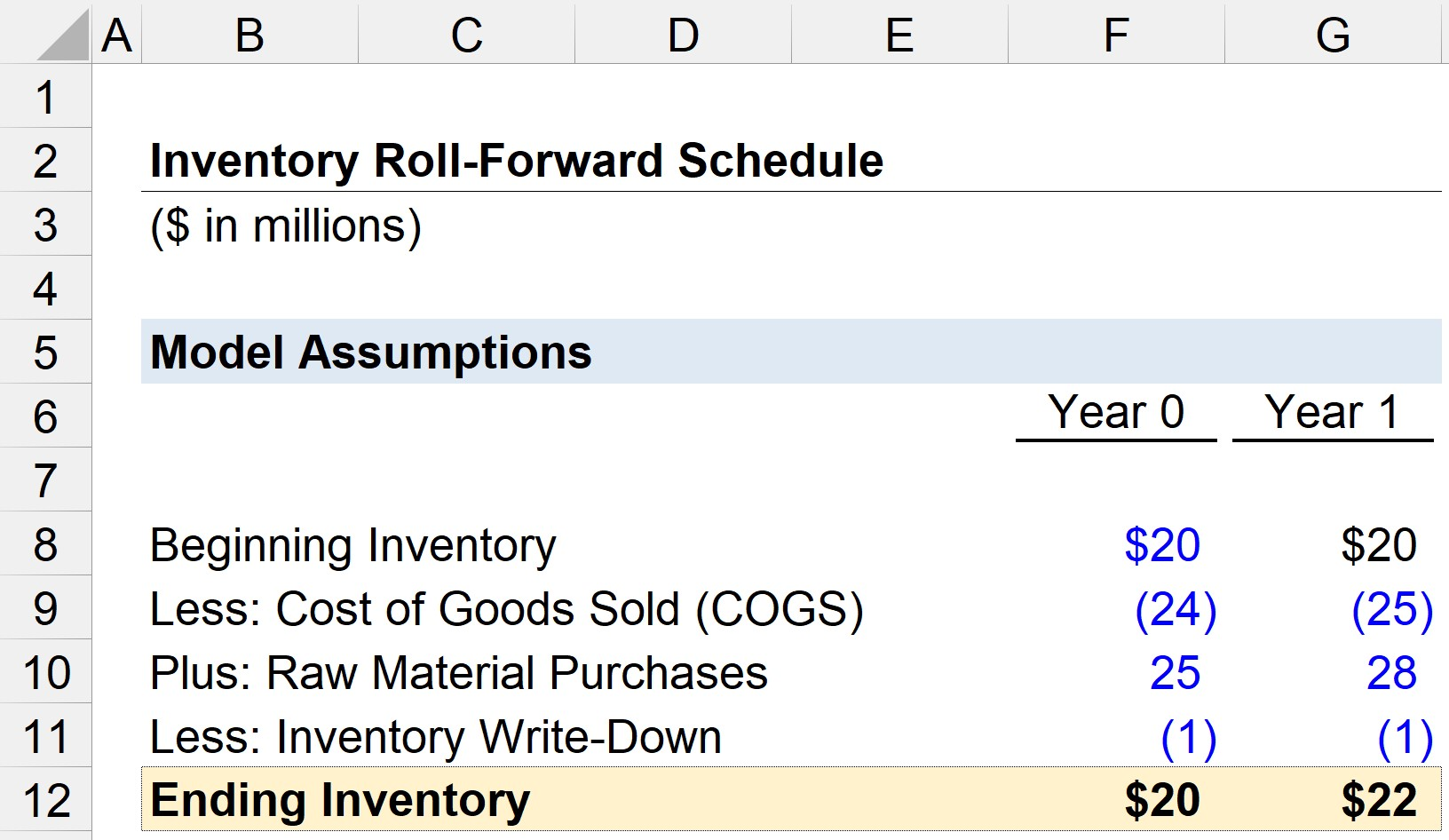# Inventory

Guide to Understanding the Concept of Inventory• What is the definition of inventory?
• What are the four different types of inventory?
• Which different methods of inventory accounting are commonly utilized?
• How is inventory recorded on the financial statements ?

## Types of Inventory

Inventory is a term referring to a wide array of materials used in the production of goods, as well the finished goods waiting to be sold.

The four different types of inventory are raw materials, work-in-progress, finished goods (available-for-sale), and maintenance, repair, and operating supplies (MRO).

1. Raw Materials: The components and parts of material necessary in the process of creating the finished product.
2. Work-In-Progress (WIP): The unfinished products in the production process (and thus not yet ready to be sold).
3. Finished Goods (Available-for-Sale): The finished products that have completed the entire production process and are now ready to be sold to customers.
4. Maintenance, Repair, and Operating Supplies (MRO): The inventory essential to the production process but not directly built into the final product itself (e.g. the protective gloves worn by employees while manufacturing the product).

## Inventory Formula

Inventory is recorded in the current assets section of the balance sheet, since unlike fixed assets (PP&E) —which have useful lives of greater than twelve months — inventory is usually expected to be cycled out (i.e. sold) within one year.

The carrying value of a company’s inventory balance is affected by two main factors:

1. Cost of Goods Sold (COGS): On the balance sheet, inventory is reduced by COGS, whose value is dependent on the type of inventory accounting method used (e.g. FIFO, LIFO, weighted average).
2. New Inventory Purchases: As part of the normal course of business, a company must replenish its inventory as needed by purchasing new raw materials.
###### Inventory Formula
• Ending Inventory = Beginning Inventory – COGS + Raw Material Purchases

## Recording Inventories on Financial Statements

There is no inventory line item on the income statement, but it gets indirectly captured in the cost of goods sold (or operating expenses) — regardless of whether the corresponding inventory was purchased in the matching period, COGS always reflects a portion of the inventory that was used.

On the cash flow statement, the change in inventory is recorded in the cash from operations section, i.e. capturing the difference in carrying values of the inventory balance between the beginning of period and end of period.

• Increase in Inventory → Cash Outflow (”Use”)
• Decrease in Inventory → Cash Inflow (”Source”)

By ordering materials on an as-needed basis and minimizing the time that inventory remains on shelves until being sold, the company has less free cash flow (FCFs) tied up in operations (and thus more cash available to execute other initiatives).

###### Inventory Write-Down vs Write-Off
• Write-Downs: In an inventory write-down, an adjustment is made for impairment, which means that the fair market value (FMV) of the asset has declined below its book value.
• Write-Offs: The inventory still retains some value post-write down, but in a write-off, the asset’s value is wiped out (i.e. reduced to zero) and is completely removed from the balance sheet.

## LIFO vs. FIFO Inventory Accounting Methods

LIFO and FIFO are the top two most common inventory accounting methods used to record the value of inventories sold in a given period.

1. Last In, First Out (LIFO): Under LIFO accounting, the most recently purchased inventories are assumed to be the ones to sold first.
2. First In, First Out (“FIFO”): Under FIFO accounting, the goods that were purchased earlier are recognized first and expensed on the income statement first.

The impact on net income depends on how the price of inventory costs has changed over time.

Last In, First Out (LIFO) First In, First Out (FIFO)
Rising Inventory Costs
• If inventory costs have been increasing, COGS for earlier periods will be higher under LIFO since the recent, pricier purchases are assumed to be sold first
• The higher COGS results in a reduced net income for those earlier periods.
• If inventory costs are rising, using FIFO would cause the recorded COGS to be lower in the near term.
• The cheaper inventory costs are recognized first, so net income is higher in earlier periods.
Declining Inventory Costs
• If inventory costs have been declining, COGS would be lower under LIFO in earlier periods.
• In effect, net income for earlier periods would be higher because the lower inventory costs are recognized.
• If inventory costs have been decreasing, COGS would be higher under FIFO as the recognized inventory costs are the older, more expensive ones.
• The ending impact is a reduced net income for the current period.

The weighted-average cost method is the third most widely used inventory accounting method after LIFO and FIFO.

Under the weighted-average method, the inventory costs recognized are based on a weighted average calculation, in which the total production costs are added and then divided by the total number of items produced in the period.

Since each product cost is treated as equivalent and the inventory costs are “spread out” equally in even amounts, the date of purchase or production is ignored.

Hence, the method is often criticized as too simplistic of a compromise between LIFO and FIFO, especially if the product characteristics (e.g. prices) have undergone significant changes over time.

Under U.S. GAAP, FIFO, LIFO, and the Weighted Average Method are all permitted, but note that IFRS does not allow LIFO.

## Inventory Management — KPI Ratios

Days inventory outstanding (DIO) measures the average number of days it takes for a company to sell off its inventory. Companies aim to optimize their DIO by quickly selling their inventory.

• DIH = (Inventory / COGS) x 365 Days

The inventory turnover ratio measures how often a company has sold and replaced its inventory balance throughout a specified period (i.e. the number of times inventory was “turned over”).

• Inventory Turnover = COGS / Average Inventory Balance

The DIO metric can be calculated using inventory turnover (and vice versa).

For instance, if we assume that a company’s DIO is 50 days, we could calculate the inventory turnover by dividing 365 days by the DIO.

• Inventory Turnover = 365 Days / 50 Days = 7.3x

And with the 7.3x ratio, we can calculate the DIO as 50 days, which was our original assumption.

• DIO = 365 Days / 7.3x = 50 Days

When interpreting the KPIs, the following rules are generally true:

• Low DIO + High Inventory Turnover → Efficient Inventory Management
• High DIO + Low Inventory Turnover → Inefficient Inventory Management
###### Projecting Inventory

In most cases, a company’s inventory grows in line with COGS, especially since DIO tends to decline over time as most companies become more efficient at selling their inventory as they mature.

DIO is usually first calculated for historical periods so that historical trends or an average of the past couple of periods can be used to guide future assumptions.

Under this method, the projected inventory balance is equal to the DIO assumption divided by 365, which is then multiplied by the forecasted COGS amount.

The efficient management of inventory enables companies to minimize costs (and increase profit margins).

When projecting inventory, either days inventory outstanding (DIO) or the inventory turnover is usually used as the driver.

## Inventory Calculator — Excel Template

We’ll now move to a modeling exercise, which you can access by filling out the form below.Submitting ...

## Inventory Roll-Forward Schedule Example

Suppose we are building a roll-forward schedule of a company’s inventory balance.

Starting off, we’ll assume that the beginning of period (BOP) inventory balance is \$20 million, which is impacted by the following factors:

• Cost of Goods (COGS) = \$24 million
• Raw Material Purchases = \$25 million
• Inventory Write-Down = \$1 million

COGS and the write-down represent reductions to the inventory balance, whereas the purchase of raw materials increases the carrying value.

• Ending Inventory = \$20 million – \$24 million + \$25 million – \$1 million = \$20 million

The net change in inventory during Year 0 was zero, as the reductions were offset by the purchases of new raw materials.

For Year 1, the beginning balance is first linked to the ending balance of the prior year, \$20 million — which will be affected by the following changes in the period.

• Cost of Goods (COGS) = \$25 million
• Raw Material Purchases = \$28 million
• Inventory Write-Down = \$1 million

Using the same equation as before, we arrive at an ending balance of \$22 million in Year 1.

• Ending Inventory = \$20 million – \$25 million + \$28 million – \$1 million = \$22 millionStep-by-Step Online Course

#### Everything You Need To Master Financial Modeling

Enroll in The Premium Package: Learn Financial Statement Modeling, DCF, M&A, LBO and Comps. The same training program used at top investment banks.Inline FeedbacksLearn Financial Modeling Online

Everything you need to master financial and valuation modeling: 3-Statement Modeling, DCF, Comps, M&A and LBO.

X

The Wall Street Prep Quicklesson Series

7 Free Financial Modeling Lessons

Get instant access to video lessons taught by experienced investment bankers. Learn financial statement modeling, DCF, M&A, LBO, Comps and Excel shortcuts.